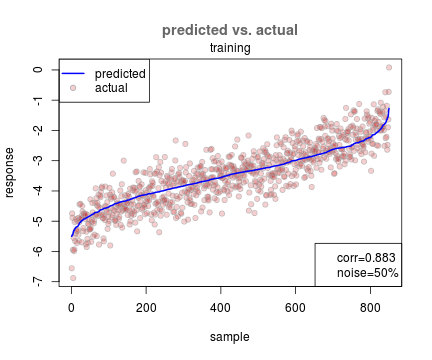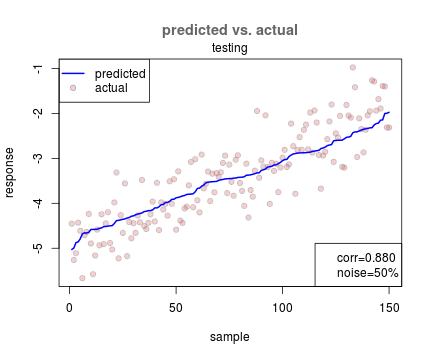## Saturday, October 27, 2012

### Feature selection and linear modeling

...being a test of the Knitr document generation tool for R.

``````library(glmnet)
``````

Let's give the glmnet package a little workout. We'll generate data for a bunch of features, some of which yield a response. Many of the features are unrelated to the response. Of course, we'll inject some noise into the data, too.

``````generate.data <- function(n=1000, m=5,
sig.features=1:5, noise.level=0.10) {

# create bogus feature matrix
features <- matrix(runif(n*m), nrow=n, ncol=m)
rownames(features) <- sprintf("ex%04d",seq(n))
colnames(features) <- sprintf("feat%04d",seq(m))

# generate random model parameters
intercept <- rnorm(1)
coefs <- rnorm(length(sig.features))
names(coefs) <- colnames(features)[sig.features]

# create response data
response <- features[,sig.features] %*% coefs
+ intercept
+ rnorm(n, sd=noise.level)

# return generated data
list(n=n, m=m,
sig.features=sig.features,
noise.level=noise.level,
features=features,
params=list(intercept=intercept, coefs=coefs),
response=response)
}
``````

A function to check correspondence between true coefficients and fit cofficients:

``````## return a data.frame containing all non-zero fit
## coefficients along with the true values
compare.coefs <- function(data, fit) {
coefs <- data\$params\$coefs
intercept <- data\$params\$intercept
merge(
data.frame(
feature.name=c('(Intercept)', names(coefs)),
param=c(`(Intercept)`=intercept, coefs)),
subset(
data.frame(
feature.name=rownames(coef(fit)),
coef=coef(fit)[,1]),
coef!=0),
all=TRUE)
}
``````

Make predicted vs actual plots

``````plot.predicted.vs.actual <-
function(data, predicted,
actual, noise.level, label=NULL) {

corr <- cor(predicted, actual)
order.by.predicted <- order(predicted)

##  create a plot of predicted vs actual
plot(actual[order.by.predicted],
pch=21, col="#aaaaaaaa", bg="#cc000030",
ylab="response", xlab="sample")

title(main="predicted vs. actual",
col.main="#666666")

lines(predicted[order.by.predicted],
col='blue', lwd=2)

legend("topleft", pch=c(NA, 21), lwd=c(2,NA),
col=c("blue", "#aaaaaa"),
pt.bg=c(NA,"#cc000030"),
legend=c('predicted','actual'))

legend("bottomright",
legend=c(
sprintf('corr=%0.3f', corr),
if (abs(noise.level) >= 2.0)
sprintf('noise=%0.1fx', noise.level)
else
sprintf('noise=%0.0f%%', noise.level*100)))
}
``````

Generate data

``````n <- 1000
noise.level <- 0.50
data <- generate.data(n, m=20,
sig.features=1:5, noise.level)
``````

Select training and testing sets

``````train <- sample.int(n, n*0.85)
test <- setdiff(seq(n), train)
``````

Fit model using elastic net.

``````fit <- cv.glmnet(data\$features[train,],
data\$response[train, drop=FALSE],
alpha=0.7)
compare.coefs(data, fit)
``````
``````  feature.name   param     coef
1  (Intercept) -2.2119 -2.32176
2     feat0001 -1.4536 -1.23601
3     feat0002  1.2987  1.12962
4     feat0003 -0.6622 -0.56728
5     feat0004  0.8445  0.70143
6     feat0005 -2.4279 -2.25502
7     feat0013      NA -0.04111
``````

Elastic net estimates the true parameters pretty well. It also gives a small weight to a spurious predictor. With 1000 features to choose from, it's not unlikely that one will look like a predictor by chance.

For some reason, cv.glmnet will cross validate over a range of lambda values, but doesn't do the same for alpha. Tweaking alpha might be help eliminate that extra coefficient.

Check our ability to model training data

``````p <- predict(fit, data\$features[train,])
plot.predicted.vs.actual(
data\$features[train,], p,
data\$response[train,],
noise.level, "training")
``````Correlation with training data

``````cor(p, data\$response[train,])
``````
``````    [,1]
1 0.8831
``````

Check our ability to predict test data

``````p <- predict(fit, data\$features[test,])
plot.predicted.vs.actual(
data\$features[test,], p,
data\$response[test,],
noise.level, "testing")
``````Correlation with testing data

``````cor(p, data\$response[test,])
``````
``````    [,1]
1 0.8799
``````

Created with the help of the excellent package Knitr. This document is also published at http://rpubs.com/cbare/2341.

1.Hi Chris,
There seem to be some problem in the code of your post, causing it to not be read properly in r-bloggers.
I've removed your post from it. If you are interested, you can republish your post (after removing possible problems in the HTML of the post), and I hope it would then load properly on r-bloggers.

With regards,
Tal

2.Hi Tal,

I hope I didn't break r-bloggers. Sorry for the hassle. I suppose some might say it was rash to copy and paste all the output from knitr right into blogger. I went back and removed some unnecessary stuff, like the extra <html> tag. That might make it more friendly.

- Chris